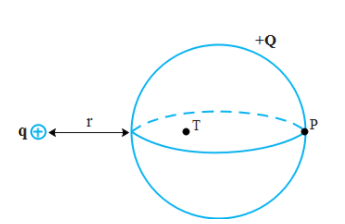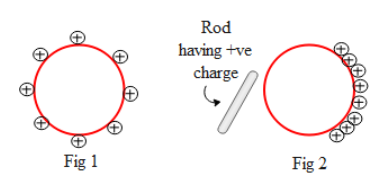QuestionAnswers

# A hollow conducting sphere has a net charge of +Q. A smaller point charge +q is located at a distance r from the left side of the hollow sphere. Point P is located on the right side of the hollow sphere and point T is located somewhere on the inside of the hollow sphere not necessarily at the centre. What happens to charge density on the hollow sphere at point P and to the electric field at point T as the charge +q is brought closer to the hollow sphere?a) The charge density at P increases and the electric field at T remains zerob) The charge density at P remains zero and the electric field at T remains zeroc) The charge density at P increases. The electric field at T increases in magnitude and always points to the rightd) The charge density at P increases and the electric field at T remains zeroe) the charge density at P remains constant but non zero. The electric field at T remains constant, but points to the leftNow let us analyze the electric field at point T. For that let us say we enclose a Gaussian surface enclosing the inside the sphere such that the point T passes through the Gaussian surface. Hence by gauss law i.e. $\text{E}\text{. }\!\!\Delta\!\!\text{ S=}\dfrac{\text{q}}{{{\in }_{\circ }}}$ where E is the electric field on the Gaussian surface, $\Delta S$ is the surface area of the Gaussian surface, q is the charge enclosed within the surface and ${{\in }_{\circ }}$is the permittivity of free space. There is no charge present within the sphere. Hence q enclosed is zero which implies the electric field on the Gaussian surface is zero. This implies that the electric field at point P is also zero since point P lies on the Gaussian surface.